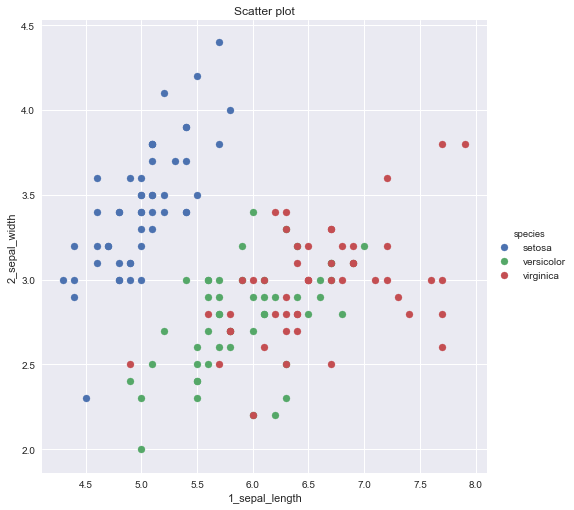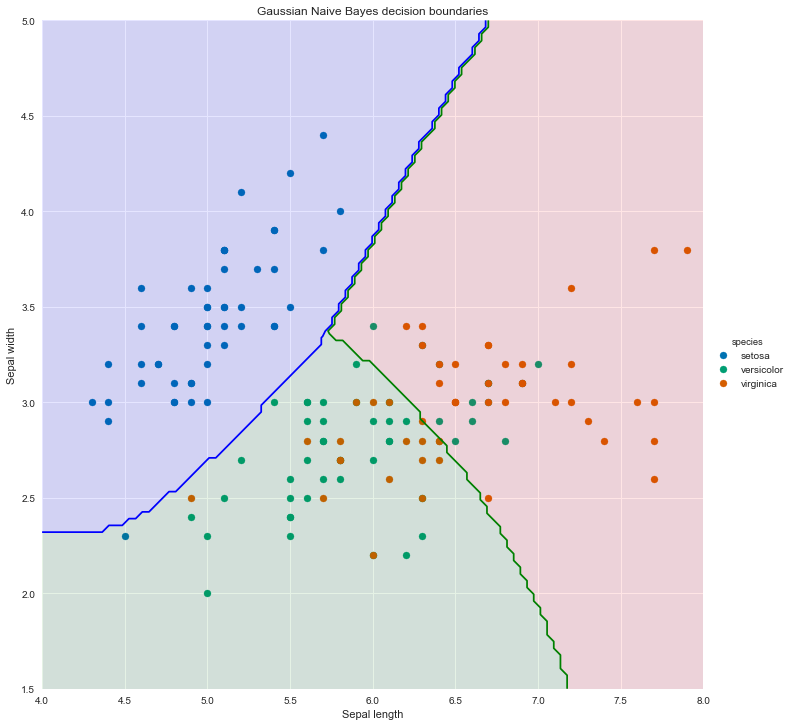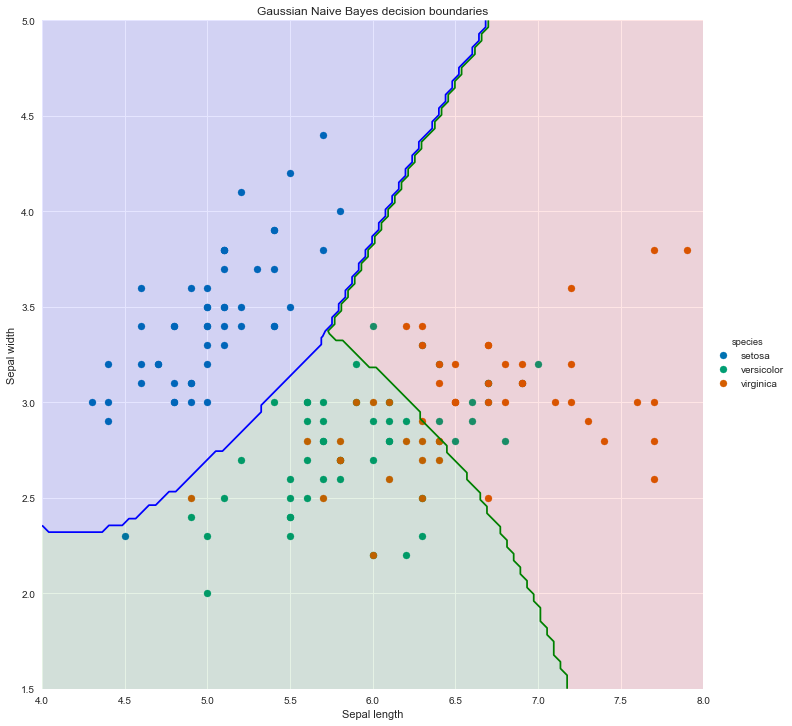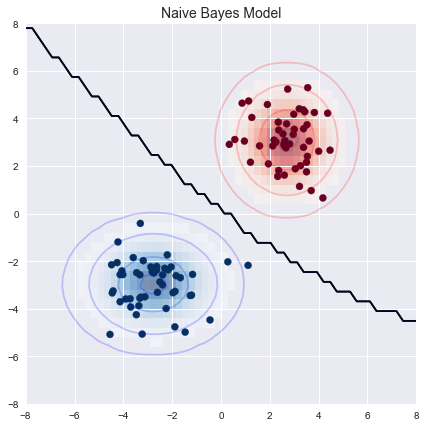# Naive Bayes¶

In this short notebook, we will re-use the Iris dataset example and implement instead a Gaussian Naive Bayes classifier using pandas, numpy and scipy.stats libraries. Results are then compared to the Sklearn implementation as a sanity check.

Note that the parameter estimates are obtained using built-in pandas functions, which greatly simplify the implementation, but may lead to slightly different results due to the way variances and covariances are calculated. (i.e. with or without bias correction)

# Theory and background¶

This is a technique that has remained popular over the years, despite its name. It is especially appropriate when the dimension $p$ of the feature space is high, making density estimation unattractive. The naive Bayes model assumes that given a class label, the features are independent

While this assumption is generally not true, it simplifies the estimation dramatically:

• The individual class-conditional marginal densities can be estimated separately
• If a component is discrete, an appropriate histogram estimate can be used. This provides a way of mixing variable types in a feature vector

### Original idea: the Bayes classifier¶

The Bayes classifier aims to estimate $\hat p(y | \vec x)$ using:

$$\hat p(y| \vec x) = \frac{p(y \cap \vec x)}{p(x)}$$

This can be estimated using the MLE method, assuming $y$ is discrete

$$\hat p(y | \vec x) = \frac{\sum_{i = 1}^m \mathcal{I}(\vec y^{(i)} = y \cap \vec x^{(i)} = \vec x) }{\sum_{i = 1}^m \mathcal{I} (\vec x^{(i)} = \vec x)}$$

However, there is a big problem with this method. The MLE estimate is only good if there are many training vectors with the same identical features as $\vec x$. In high dimensional spaces or with continuous $\vec x$ this never happens and the numerator and denominator both tend to zero.

### Naive assumption¶

To get around this issue, we make use of a naive assumption and the Bayes trick. These turn out to be powerful ones.

Use Bayes rule to rewrite the equation and recall that estimating $p(y)$ and $p(\vec x | y)$ is called \textbf{generative learning}.

$$p(y| \vec x) = \frac{p(y \cap \vec x)}{p(x)} = \frac{p(\vec x | y) p(y)}{p(\vec x)}$$

Estimating $p(y)$ is easy, if Y takes on discrete binary values, we simply need to count how many times we observe each class

$$p(y = x) = \frac{\sum_{i=1}^m \mathcal{I}(y^{(i)} = c)}{m} = \hat \pi_c$$

Estimating $p(\vec x | y)$ is not easy, and requires the naive assumption that \textit{feature values are independent given the label}. This is a very bold assumption, as is illustrated by the following example.

Imagine that we are building a Naive Bayes spam classifier, where the data are words in an email and the labels are spam vs not spam. The Naive Bayes assumption implies that words in an email are conditionally independent given that we know that an email is spam or not spam. Mathematically, if $\vec x \in R^p$ we get

\begin{aligned} p(\vec x | y) & = p(x_1 | y) \ p(x_2 | y, x_1) \ p(x_3 | y, x_1, x_2) \ ... \ p(x_p | y, x_1,...,x_{p-1}) & \text{Chain rule of probability} \\ \\ & = p(x_1|y) \ p(x_2|y) \ ... \ p(x_p | y) & \text{Conditional independence} \\ \\ & = \prod_{\alpha = 1}^p p(x_\alpha | y) & \text{Compact notation} \end{aligned}

As usual, the Bayes Classifier will predict the class for which the posterior probability is the greatest:

\begin{aligned} h(\vec x) & = argmax_y p(y | \vec x) \\ & = argmax_y \frac{p(\vec x | y) p(y)}{p(\vec x)} \\ & = argmax_y p(\vec x | y) p(y) & \text{$P(\vec x)$ does not depend on $y$} \\ & = argmax_y \prod_{\alpha = 1}^p p(x_\alpha | y) p(y) & \text{Naive assumption} \\ & = argmax_y \sum_{\alpha = 1}^p \log(p(x_\alpha| y)) + \log p(y) &\text{Log is monotonic} \end{aligned}

Note that we don't actually need to compute the probability, but can instead maximize the objective of the last two lines of the equation above as these will be proportional to the posterior probability. This makes it easier to calculate as $p(x)$ may not be known.

#### Estimating $p(\vec x | y)$}¶

Now that we have an objective function, we can use it to make estimations of $p(y | \vec x)$ based on three different probabilistic assumptions which will depend on the structure of the problem and the dataset.

## Continuous features¶

When feature $x_\alpha \in \mathcal{R}$ take on real values, we can use a Gaussian distribution

$$p(x_\alpha | y = c) \sim \mathcal{N}(\mu_{\alpha c}, \sigma^2_{\alpha c}) = \phi(x | \mu_{\alpha c}, \sigma^2_{\alpha c} )$$

where we assume that each feature $\alpha$ comes from a class-conditional, univariate Gaussian distribution. Other specifications can be used.

Parameters are estimated as

$$\mu_{\alpha c} = \frac{1}{m_c} \sum_{i = 1}^m \mathcal{I}(y^{(i)} = c) x^{(i)}_\alpha$$ $$\sigma^2_{\alpha c} = \frac{1}{m_c} \sum_{i = 1}^m \mathcal{I}(y^{(i)} = c) (x^{(i)}_\alpha - \mu_{\alpha c})^2$$ $$m_c = \sum_{i = 1}^m \mathcal{I}(y^{(i)} = c)$$ $$p(y = c) = \frac{1}{m} \sum_{i = 1}^m \mathcal{I}(y^{(i)} = c)$$

### An example in 2D using the Iris data set¶

Let's consider a simple example using the Iris data set:

• There are 3 class labels: Setosa, Versicolor, Virginica which we label as $y \in \{ 0,1,2\}$
• There are two explanatory variables (features): $X_1:$ sepal length and $X_2:$ sepal width.

For each feature, we calculate the estimated class mean, class variance and prior probability

• Means: $\mu_{x_1 | y = 0}, \mu_{x_1 | y = 1}, \mu_{x_1 | y = 2}$ and $\mu_{x_2 | y = 0}, \mu_{x_2 | y = 1}, \mu_{x_2 | y = 2}$
• Variance: $\sigma^2_{x_1 | y = 0}, \sigma^2_{x_1 | y = 1}, \sigma^2_{x_1 | y = 2}$ and $\sigma^2_{x_2 | y = 0}, \sigma^2_{x_2 | y = 1}, \sigma^2_{x_2 | y = 2}$
• Prior: $p(y = 0), p(y = 1), p(y = 2)$

For any point $(x_1, x_2)$ we compute the Gaussian Naive Bayes objective function (i.e. the one we are trying to maximize) for each class :

\begin{aligned} h(\vec x) &= argmax_y \prod_{\alpha = 1}^2 p(x_\alpha | y) p(y) \\ & = argmax_y \{ p(x_1 | y ) p(y ) \cdot p(x_2 | y ) p(y ) \} \\ & = argmax_y \{ \phi(x_1 | \mu_{x_1 | y}, \sigma^2_{x_1 | y } ) p(y ) \cdot \{ \phi(x_2 | \mu_{x_2 | y}, \sigma^2_{x_2 | y } ) p(y ) \} \end{aligned}

Where $\phi(x_1 | \mu_{x_1 | y}, \sigma^2_{x_1 | y } )$ is the PDF of a Gaussian univariate distribution with parameters $\mu_{x_1 | y}, \sigma^2_{x_1 | y }$. Repeat this calculation for each class, and then predict the class which has the highest value.

### Libraries¶

In :
import numpy as np
import pandas as pd
from matplotlib import pyplot as plt
import matplotlib.colors as colors
import seaborn as sns
import itertools
from scipy.stats import norm
import scipy.stats
from sklearn.naive_bayes import GaussianNB

%matplotlib inline
sns.set()


## Iris dataset and scatter plot¶

### Sepal length vs sepal width¶

In :
#Load the data set
iris = iris.rename(index = str, columns = {'sepal_length':'1_sepal_length','sepal_width':'2_sepal_width', 'petal_length':'3_petal_length', 'petal_width':'4_petal_width'})

#Plot the scatter of sepal length vs sepal width
sns.FacetGrid(iris, hue="species", size=7) .map(plt.scatter,"1_sepal_length", "2_sepal_width", )  .add_legend()
plt.title('Scatter plot')
df1 = iris[["1_sepal_length", "2_sepal_width",'species']]# Gaussian Naive Bayes: Numpy implementation¶

### Numpy implementation¶

In :
def predict_NB_gaussian_class(X,mu_list,std_list,pi_list):
#Returns the class for which the Gaussian Naive Bayes objective function has greatest value
scores_list = []
classes = len(mu_list)

for p in range(classes):
score = (norm.pdf(x = X, loc = mu_list[p], scale = std_list[p] )
* norm.pdf(x = X, loc = mu_list[p], scale = std_list[p] )
* pi_list[p])
scores_list.append(score)

return np.argmax(scores_list)

def predict_Bayes_class(X,mu_list,sigma_list):
#Returns the predicted class from an optimal bayes classifier - distributions must be known
scores_list = []
classes = len(mu_list)

for p in range(classes):
score = scipy.stats.multivariate_normal.pdf(X, mean=mu_list[p], cov=sigma_list[p])
scores_list.append(score)

return np.argmax(scores_list)


### Plotting the decision boundaries¶

In :
#Estimating the parameters
mu_list = np.split(df1.groupby('species').mean().values,[1,2])
std_list = np.split(df1.groupby('species').std().values,[1,2], axis = 0)
pi_list = df1.iloc[:,2].value_counts().values / len(df1)

# Our 2-dimensional distribution will be over variables X and Y
N = 100
X = np.linspace(4, 8, N)
Y = np.linspace(1.5, 5, N)
X, Y = np.meshgrid(X, Y)

#fig = plt.figure(figsize = (10,10))
#ax = fig.gca()
color_list = ['Blues','Greens','Reds']
my_norm = colors.Normalize(vmin=-1.,vmax=1.)

g = sns.FacetGrid(iris, hue="species", size=10, palette = 'colorblind') .map(plt.scatter, "1_sepal_length", "2_sepal_width",)  .add_legend()
my_ax = g.ax

#Computing the predicted class function for each value on the grid
zz = np.array(  [predict_NB_gaussian_class( np.array([xx,yy]).reshape(-1,1), mu_list, std_list, pi_list)
for xx, yy in zip(np.ravel(X), np.ravel(Y)) ] )

#Reshaping the predicted class into the meshgrid shape
Z = zz.reshape(X.shape)

#Plot the filled and boundary contours
my_ax.contourf( X, Y, Z, 2, alpha = .1, colors = ('blue','green','red'))
my_ax.contour( X, Y, Z, 2, alpha = 1, colors = ('blue','green','red'))

my_ax.set_xlabel('Sepal length')
my_ax.set_ylabel('Sepal width')
my_ax.set_title('Gaussian Naive Bayes decision boundaries')

plt.show()# Gaussian Naive Bayes: Sklearn implementation¶

In :
from sklearn.naive_bayes import GaussianNB

#Setup X and y data
X_data = df1.iloc[:,0:2]
y_labels = df1.iloc[:,2].replace({'setosa':0,'versicolor':1,'virginica':2}).copy()

#Fit model
model_sk = GaussianNB(priors = None)
model_sk.fit(X_data,y_labels)

# Our 2-dimensional classifier will be over variables X and Y
N = 100
X = np.linspace(4, 8, N)
Y = np.linspace(1.5, 5, N)
X, Y = np.meshgrid(X, Y)

#fig = plt.figure(figsize = (10,10))
#ax = fig.gca()
color_list = ['Blues','Greens','Reds']
my_norm = colors.Normalize(vmin=-1.,vmax=1.)

g = sns.FacetGrid(iris, hue="species", size=10, palette = 'colorblind') .map(plt.scatter, "1_sepal_length", "2_sepal_width",)  .add_legend()
my_ax = g.ax

#Computing the predicted class function for each value on the grid
zz = np.array(  [model_sk.predict( [[xx,yy]]) for xx, yy in zip(np.ravel(X), np.ravel(Y)) ] )

#Reshaping the predicted class into the meshgrid shape
Z = zz.reshape(X.shape)

#Plot the filled and boundary contours
my_ax.contourf( X, Y, Z, 2, alpha = .1, colors = ('blue','green','red'))
my_ax.contour( X, Y, Z, 2, alpha = 1, colors = ('blue','green','red'))

my_ax.set_xlabel('Sepal length')
my_ax.set_ylabel('Sepal width')
my_ax.set_title('Gaussian Naive Bayes decision boundaries')

plt.show()## Comparing the Accuracy of both implementations¶

In :
#Numpy accuracy
y_pred = np.array(  [predict_NB_gaussian_class( np.array([xx,yy]).reshape(-1,1), mu_list, std_list, pi_list)
for xx, yy in zip(np.ravel(X_data.values[:,0]), np.ravel(X_data.values[:,1])) ] )
display(np.mean(y_pred == y_labels))

#Sklearn accuracy
display(model_sk.score(X_data,y_labels))

0.78
0.78

## Miscellaneous¶

In :
from sklearn.datasets import make_blobs
X, y = make_blobs(100, 2, centers=[[3,3],[-3,-3]], random_state=2, cluster_std=[1,1])

fig, ax = plt.subplots(figsize = (7,7))

ax.scatter(X[:, 0], X[:, 1], c=y, s=50, cmap='RdBu')
ax.set_title('Naive Bayes Model', size=14)

xlim = (-8, 8)
ylim = (-8, 8)

xg = np.linspace(xlim, xlim, 60)
yg = np.linspace(ylim, ylim, 40)
xx, yy = np.meshgrid(xg, yg)
Xgrid = np.vstack([xx.ravel(), yy.ravel()]).T

#Fit model
model_sk_1 = GaussianNB(priors = None)
model_sk_1.fit(X,y)

for label, color in enumerate(['red', 'blue']):
P = np.exp(-0.5 * (Xgrid - mu) ** 2 / std ** 2).prod(1)
Pm = np.ma.masked_array(P, P < 0.03)
ax.pcolorfast(xg, yg, Pm.reshape(xx.shape), alpha=0.5,
cmap=color.title() + 's')
ax.contour(xx, yy, P.reshape(xx.shape),
levels=[0.01, 0.1, 0.5, 0.9],
colors=color, alpha=0.2)
Xgrid_Pred = model_sk_1.predict(Xgrid)
ax.contour(xx, yy, Xgrid_Pred.reshape(xx.shape),1,color = 'red')

ax.set(xlim=xlim, ylim=ylim)

/anaconda3/lib/python3.6/site-packages/matplotlib/contour.py:967: UserWarning: The following kwargs were not used by contour: 'color'
s)

Out:
[(-8, 8), (-8, 8)]